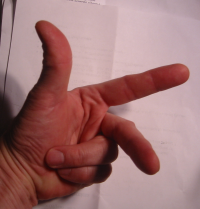## Representations of the Quaternions

The various possible multiplication tables of octonions, twisted octonions, and sedenions depend directly on a few basic properties of multiplication of quaternions:
• there are 4 basis elements:
• e0 (equivalent to the unit element of the real numbers), and
• 3 other distinct "imaginary" elements e1, e2, e3 such that ei2 = -1
(historically these imaginary elements were first called i, j, k)
• the basis elements multiply according to one of the two following "multiplication tables":
e0e1e2e3
e0e0e1e2e3
e1e1-e0-e3e2
e2e2e3-e0-e1
e3e3-e2e1-e0
OR
e0e1e2e3
e0e0e1e2e3
e1e1-e0e3-e2
e2e2-e3-e0e1
e3e3e2-e1-e0
(the interrelationship between these two alternatives will be discussed below, and is important in generalizing to higher dimensions)
"Left-handed""Right-handed"
i2=j2=k2=kji=-1i2=j2=k2=ijk=-1

• an arbitrary quaternion is of the form r0e0 + r1e1 + r2e2 + r3e3, with ri real numbers
• the real coefficients ri are commutative and associative with any multiplicative combination of the basis elements,
e.g. riei((rjej)(rkek)) = rirjrk(ei(ejek))
• multiplication is distributive over addition
With these properties the multiplication of any 2 quaternions gives a unique quaternion as the product; the exact values depend on which of the two multiplication tables above ("Left-handed" vs. "Right-handed") is chosen.

Restricting attention to just the set {+ei, -ei}, 0 ≤ i ≤ 3, gives a finite group (in the case of the quaternions) or loop (nonassociative analog of a group) in higher dimensions; the essence of multiplication in the system being investigated is encapsulated in these finite loops, and most of the following presentation will focus on these finite cases. In particular, symmetry groups are simplified - in the quaternion case, actions of the permutation group on {1,2,3} and reflection of one or more axes will map a multiplication table above onto itself or the multiplication table of opposite "handedness".The "-handed" labels are a reflection of terminology in 3D geometry and physics - a system of 3D axes is termed "Left-handed" if these axes, in ascending order of index (e.g. x,y,z or i,j,k or e1, e2, e3) have the same orientation as the thumb, index finger, and middle finger of the left hand; similarly for "Right-handed", in mirror-image. Parity and chirality are concepts in physics intimately related to this notion of handedness. Note that a transformation of one table via axis inversion will give the other table. The same convention defining handedness (i.e. a triad of basis elements (ei, ej, ek) i < j < k is "Right-handed" if eiej = +ek, and "Left-handed" if eiej = -ek) will be used to define a more complex notion of parity in higher-dimensions, where axis inversion is not equivalent to handedness reversal. One further observation that will be helpful later - the indexes i, j, k in the above multiplication tables obey eiej = [+/-] ei^j (modulo sign), where '^' denotes bitwise exclusive OR (XOR).